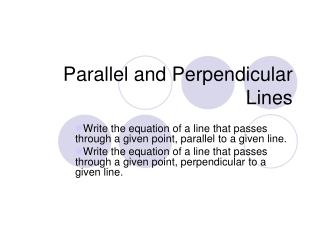DownloadDownload PresentationParallel and Perpendicular Lines

# Parallel and Perpendicular Lines

Télécharger la présentation## Parallel and Perpendicular Lines

- - - - - - - - - - - - - - - - - - - - - - - - - - - E N D - - - - - - - - - - - - - - - - - - - - - - - - - - -
##### Presentation Transcript

1. Parallel and Perpendicular Lines Write the equation of a line that passes through a given point, parallel to a given line. Write the equation of a line that passes through a given point, perpendicular to a given line.

2. Parallel lines Lines in the same plane that do not intersect are called parallel lines. Parallel lines have the same slope.

3. Perpendicular Lines Lines that intersect at right angles are called perpendicular lines. The slopes of these lines are opposite reciprocals. Opposite reciprocals???? 3…..

4. From the given equations, determine if the corresponding lines are parallel, perpendicular, or neither. y = 2x + 2 y = 4x - 2 neither 2x + 6y = 1 4x + 12y =3 parallel perpendicular

5. Write the slope intercept form of an equation for the line that passes through (12,3), and is parallel to the graph of

6. Write the slope intercept form of an equation for the line that passes through (1, -3), and is perpendicular to the graph of

7. Write the slope intercept form of an equation for the line that passes through (-6, 5), and is perpendicular to the graph of x - y= 5